# Graphing Quadratic Functions Concept A quadratic function in

• Slides: 14Graphing Quadratic Functions – Concept • A quadratic function in what we will call Standard Form is given by: • The graph of a quadratic function is called a parabola. Here is the graph of a very simple quadratic function: Table of Contents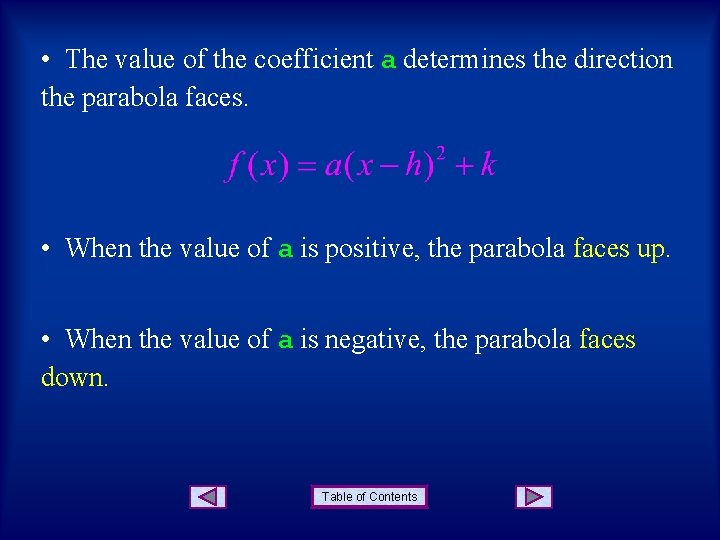• The value of the coefficient a determines the direction the parabola faces. • When the value of a is positive, the parabola faces up. • When the value of a is negative, the parabola faces down. Table of Contents• Example 1: Face Down Face Up Table of Contents• The value of the coefficient a also determines the shape of the parabola. • When |a| > 1 the parabola is narrow. • When 0 < |a| < 1 the parabola is wide. Table of Contents• The vertex of a parabola is the highest point or the lowest point on the graph of a parabola. Vertex Table of Contents• The vertex of a parabola whose function is given in standard form … … is given by V(h, k). • Example 3: The vertex is given by: Table of Contents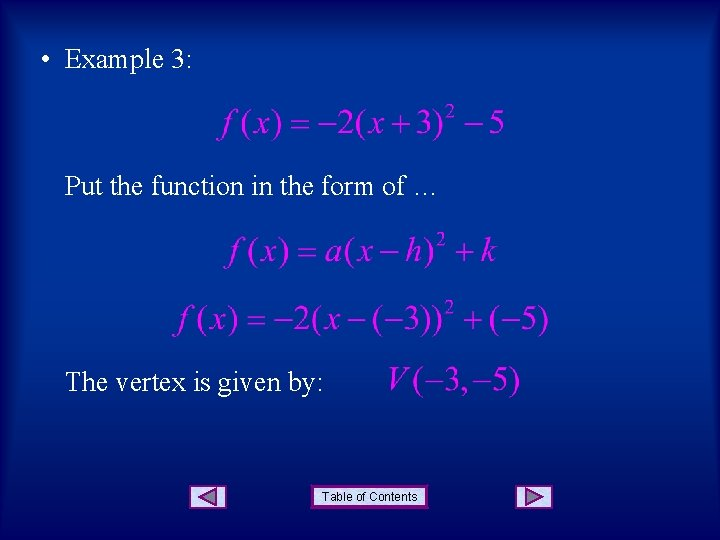• Example 3: Put the function in the form of … The vertex is given by: Table of Contents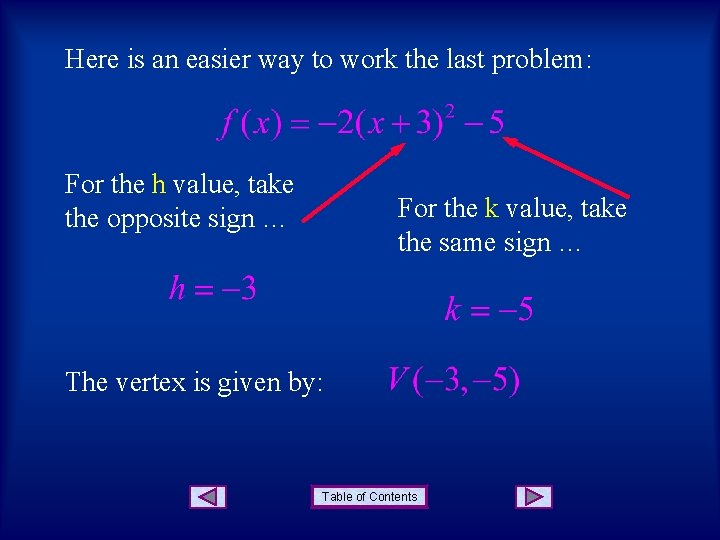Here is an easier way to work the last problem: For the h value, take the opposite sign … For the k value, take the same sign … The vertex is given by: Table of Contents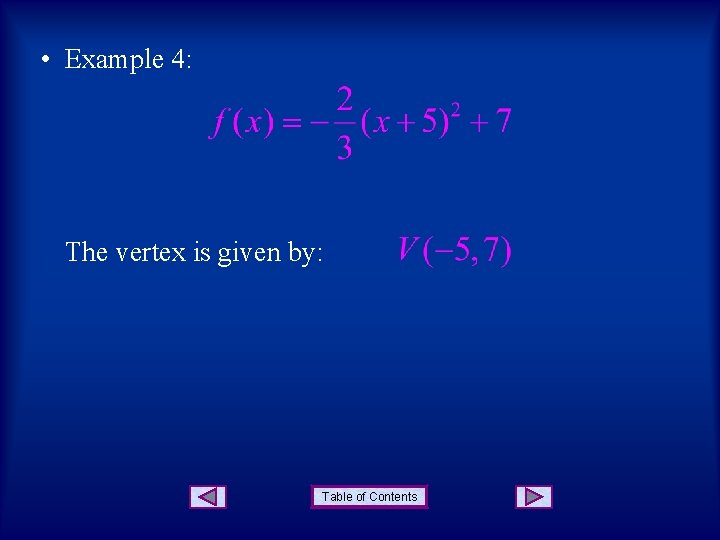• Example 4: The vertex is given by: Table of Contents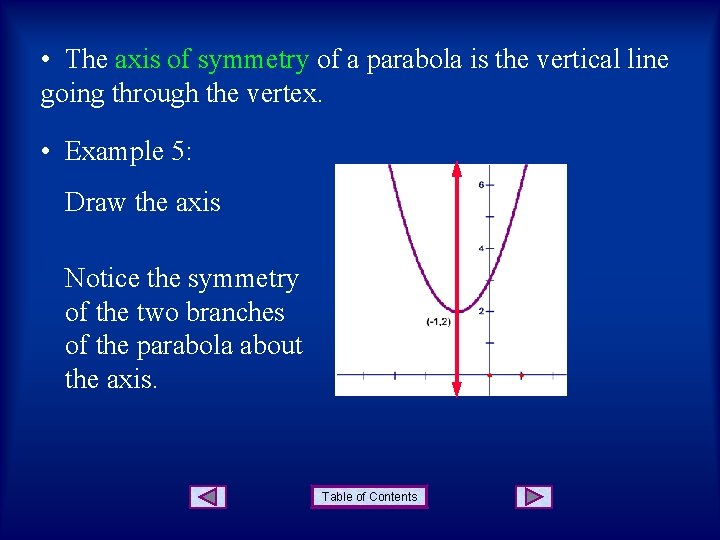• The axis of symmetry of a parabola is the vertical line going through the vertex. • Example 5: Draw the axis Notice the symmetry of the two branches of the parabola about the axis. Table of Contents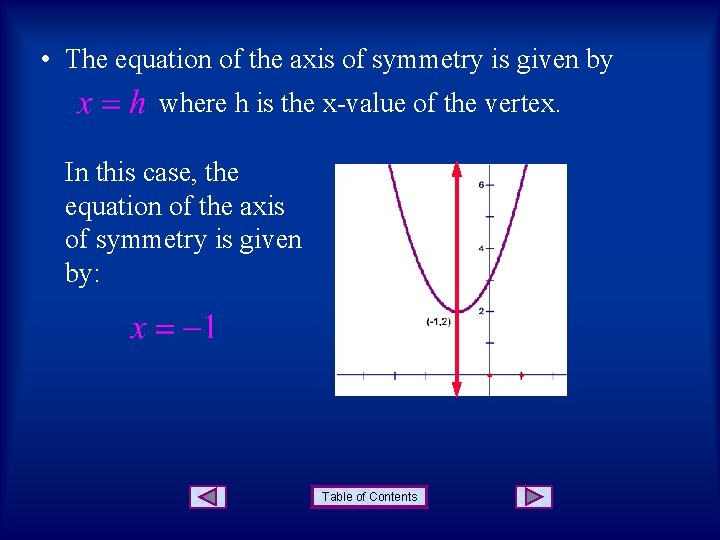• The equation of the axis of symmetry is given by where h is the x-value of the vertex. In this case, the equation of the axis of symmetry is given by: Table of ContentsSUMMARY Vertex Face Up Face Down Axis of symmetry Narrow Wide Table of Contents